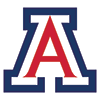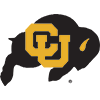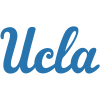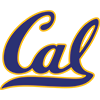DraftKings 2019 Pac-12 Odds
+200
2 to 1Oregon
28.3% implied probability

+350
3.5 to 1Arizona
18.9% implied probability

+400
4 to 1Colorado
17.0% implied probability

+700
7 to 1UCLA
10.6% implied probability

+1000
10 to 1USC
7.7% implied probability

+1400
14 to 1Arizona State
5.7% implied probability

+1400
14 to 1Stanford
5.7% implied probability

+4000
40 to 1Washington
2.1% implied probability

+5000
50 to 1Oregon State
1.7% implied probability

+10000
100 to 1California
0.8% implied probability

+10000
100 to 1Utah
0.8% implied probability

+10000
100 to 1Washington State
0.8% implied probability